# SAT Math : How to find the length of the hypotenuse of a right triangle : Pythagorean Theorem

## Example Questions

### Example Question #1 : Apply The Pythagorean Theorem To Find The Distance Between Two Points In A Coordinate System: Ccss.Math.Content.8.G.B.8

Max starts at Point A and travels 6 miles north to Point B and then 4 miles east to Point C. What is the shortest distance from Point A to Point C?

5 miles

4√2 miles

10 miles

2√13 miles

7 miles

2√13 miles

Explanation:

This can be solved with the Pythagorean Theorem.

62 + 42 = c2

52 = c2

c = √52 = 2√13

### Example Question #51 : Triangles

Which set of side lengths CANNOT correspond to a right triangle?

7, 24, 25

5, 12, 13

8, 15, 17

6, 8, 11

3, 4, 5

6, 8, 11

Explanation:

Because we are told this is a right triangle, we can use the Pythagorean Theorem, a2 + b2 = c2. You may also remember some of these as special right triangles that are good to memorize, such as 3, 4, 5.

Here, 6, 8, 11 will not be the sides to a right triangle because 62 + 82 = 102.

### Example Question #1 : Apply The Pythagorean Theorem To Find The Distance Between Two Points In A Coordinate System: Ccss.Math.Content.8.G.B.8

Angela drives 30 miles north and then 40 miles east. How far is she from where she began?

35 miles

50 miles

45 miles

60 miles

50 miles

Explanation:

By drawing Angela’s route, we can connect her end point and her start point with a straight line and will then have a right triangle. The Pythagorean theorem can be used to solve for how far she is from the starting point: a2+b2=c2, 302+402=c2, c=50. It can also be noted that Angela’s route represents a multiple of the 3-4-5 Pythagorean triple.

### Example Question #1231 : Basic Geometry

To get from his house to the hardware store, Bob must drive 3 miles to the east and then 4 miles to the north. If Bob was able to drive along a straight line directly connecting his house to the store, how far would he have to travel then?

5 miles
25 miles
15 miles
7 miles
9 miles
Explanation:

Since east and north directions are perpendicular, the possible routes Bob can take can be represented by a right triangle with sides a and b of length 3 miles and 5 miles, respectively. The hypotenuse c represents the straight line connecting his house to the store, and its length can be found using the Pythagorean theorem: c2 = 32+ 42 = 25. Since the square root of 25 is 5, the length of the hypotenuse is 5 miles.

### Example Question #91 : Geometry

A park is designed to fit within the confines of a triangular lot in the middle of a city.  The side that borders Elm street is 15 feet long. The side that borders Broad street is 23 feet long. Elm street and Broad street meet at a right angle. The third side of the park borders Popeye street, what is the length of the side of the park that borders Popeye street?

22.5 feet

17.44 feet

27.46 feet

16.05 feet

18.5 feet

27.46 feet

Explanation:

This question requires the use of Pythagorean Theorem. We are given the length of two sides of a triangle and asked to find the third. We are told that the two sides we are given meet at a right angle, this means that the missing side is the hypotenuse. So we use a+ b= c2, plugging in the two known lengths for a and b. This yields an answer of 27.46 feet.

### Example Question #31 : Triangles

A right triangle has legs of 15m and 20m. What is the length of the hypotenuse?

40m

45m

30m

35m

25m

25m

Explanation:

The Pythagorean theorem is a2 + b2 = c2, where a and b are legs of the right triangle, and c is the hypotenuse.

(15)2 + (20)2 = c2 so c2 = 625.  Take the square root to get c = 25m

### Example Question #41 : Right Triangles

Paul leaves his home and jogs 3 miles due north and 4 miles due west. If Paul could walk a straight line from his current position back to his house, how far, in miles, is Paul from home?

√14

5

7

4

25

5

Explanation:

By using the Pythagorean Theorem, we can solve for the distance “as the crow flies” from Paul to his home:

32 + 42 = x2

9 + 16 = x2

25 = x2

5 = x

### Example Question #251 : Triangles

Given a right triangle where the two legs have lengths of 3 and 4 respectively, what is the length of the hypotenuse?

25

3

4

5

9

5

Explanation:

The hypotenuse can be found using Pythagorean Theorem, which is a+ b= c2, so we plug in a = 3 and b = 4 to get c.

c2  =25, so c = 5

### Example Question #31 : Right Triangles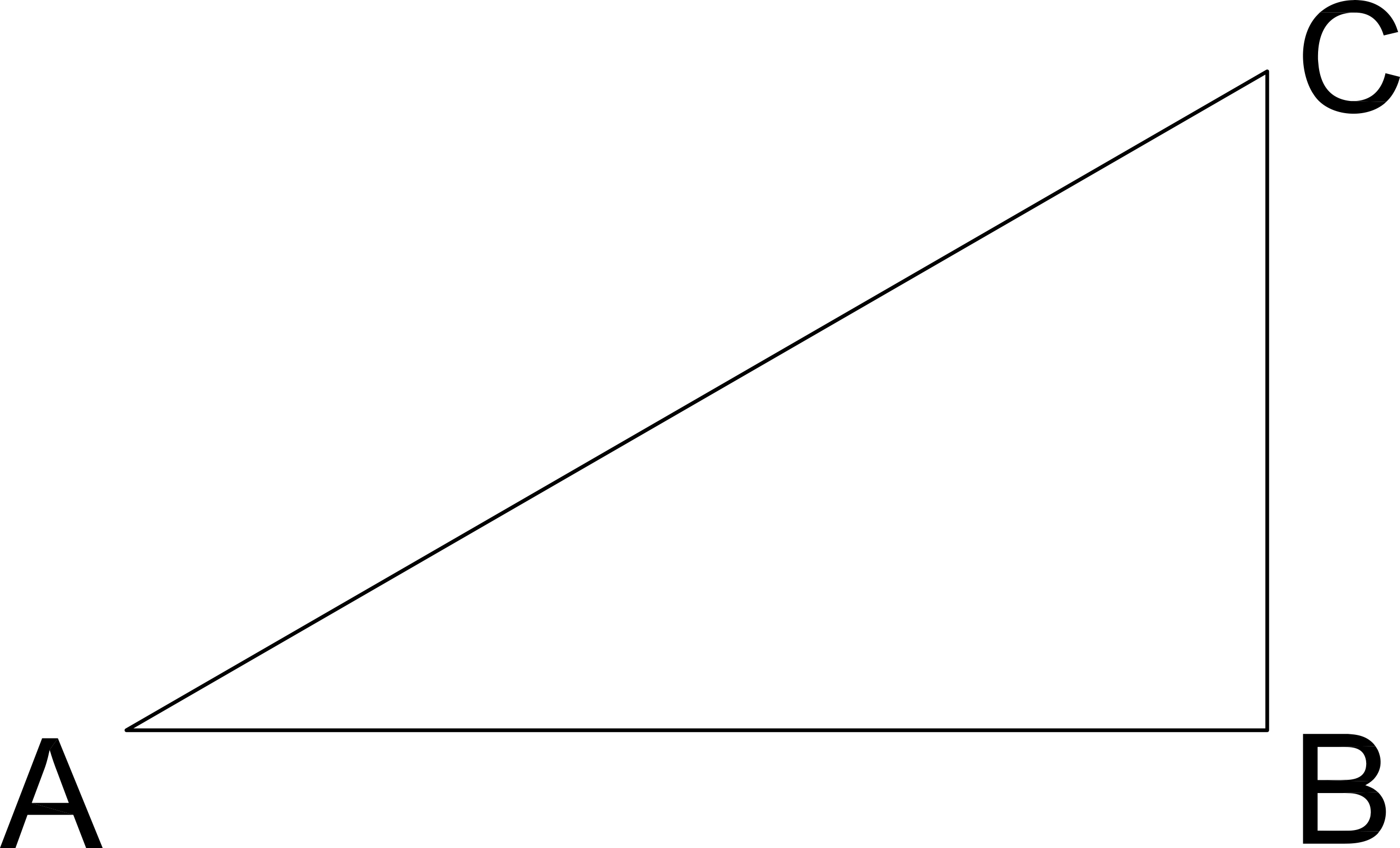Length AB = 4

Length BC = 3

If a similar triangle has a hypotenuse length of 25, what are the lengths of its two legs?

15 and 20

3 and 4

5 and 25

15 and 25

20 and 25

15 and 20

Explanation:

Similar triangles are in proportion.

Use Pythagorean Theorem to solve for AC:

Pythagorean Theorem:  AB2 + BC2 = AC2

42 + 32 = AC2

16 + 9 = AC2

25 = AC2

AC = 5

If the similar triangle's hypotenuse is 25, then the proportion of the sides is AC/25 or  5/25 or 1/5.

Two legs then are 5 times longer than AB or BC:

5 * (AB) = 5 * (4) = 20

5 * (BC) = 5 * (3) = 15

### Example Question #32 : Right Triangles

If the base of a right triangle is 5 cm long and the height of the triangle is 7 cm longer than the base, what is the length of the third side of the triangle in cm?Explanation:

Find the height of the triangle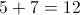Use the Pythagorean Theorem to solve for the length of the third side, or hypotenuse.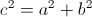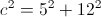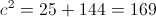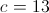### All SAT Math Resources